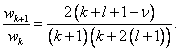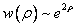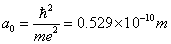# Physics 751 Homework #12

Due Wednesday December 3.

1. VerifyArgue that this leads to asymptotic behaviorfor general values of the energy.

2.is called the Bohr radius:  it is in fact the radius of the lowest orbit in Bohr’s model.

Exercise: check this.

3.  For n = 4, what is the energy?  What are the possible values of the angular momentum? What is the total degeneracy (number of possible states at this energy)?

Work out the expressions for, and then sketch, the possible n = 4  radial wave functions. Give a brief interpretation of each: how would you describe the electron’s motion, and how does the wave function indicate this?

Shankar 14.4.6: this is a Density Matrix problem: I’ll do Density Matrices on Monday.